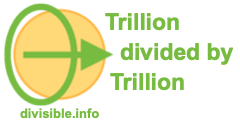Trillion divided by Trillion CalculatorWelcome to our Trillion divided by Trillion Calculator. When working with trillions, we are dealing with a lot of zeros.

This means that it's easy to make a mistake when you enter your trillion numbers into a pocket calculator. In addition, a lot of calculators may not even allow you to enter that many zeros or digits.

Use our Trillion divided by Trillion Calculator to easily divide trillions by trillions! Please enter your math problem below so we can solve it for you.

trillion divided by  trillion

Here are some examples of what our Trillion divided by Trillion Calculator can calculate for you.

What is 408 trillion divided by 60 trillion?

What is 400 trillion divided by 60 trillion?

What is 10 trillion divided by 100 trillion?

What is 1 trillion divided by 100 trillion?

What is 190 trillion divided by 98 trillion?

What is 350 trillion divided by 76 trillion?

What is 6 trillion divided by 150 trillion?